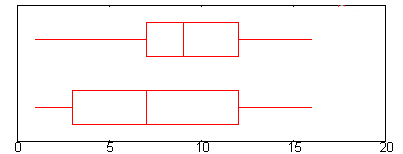# Middle Fifty in Statistics: What is it?

Share on

Statistics Definitions > What is the Middle Fifty?

## The Middle fiftyThe middle fifty is another name for the interquartile range, which is a measure of spread in statistics. The middle fifty is useful for seeing where the bulk of the values in the data set lie, and how those values are clustered around the mean. It’s commonly used to report test scores and in reporting college admissions statistics. It even has more diverse applications like analyzing asylum decisions.

### Score Reporting and College Admissions

The “middle fifty” is often used to report college admissions test scores, like SAT scores. If the score for a certain school is 1250 to 1350, it means that 25 percent of the students scores below 1250, and 25 percent scored above 1350. The bulk of the students (the middle fifty) scored between 1250 and 1350. Many universities also use the middle 50% to describe their student body. For example, Rutger’s University reports the middle fifty for admitted students’ SAT scores, ACT scores, GPA and high school percentile rank. It can assist you with applying to college; If your scores fall in the high end reported for that school, it indicates you have a very good chance of being admitted to that school based on your scores. Basically, this statistic tells you where the bulk of the student scores are.

### Calculation

The middle 50% is usually calculated by subtracting the first quartile (the 25% mark) from the third quartile (the 75% mark):
Middle 50% = Q3 – Q1.

### Use in a Normal Distribution

In a normal distribution, the first and third quartiles are at -0.67448 and +0.67448, so the calculation for the middle 50% is:
Middle 50% = 2(0.67448)*σ = 1.34896σ

## References